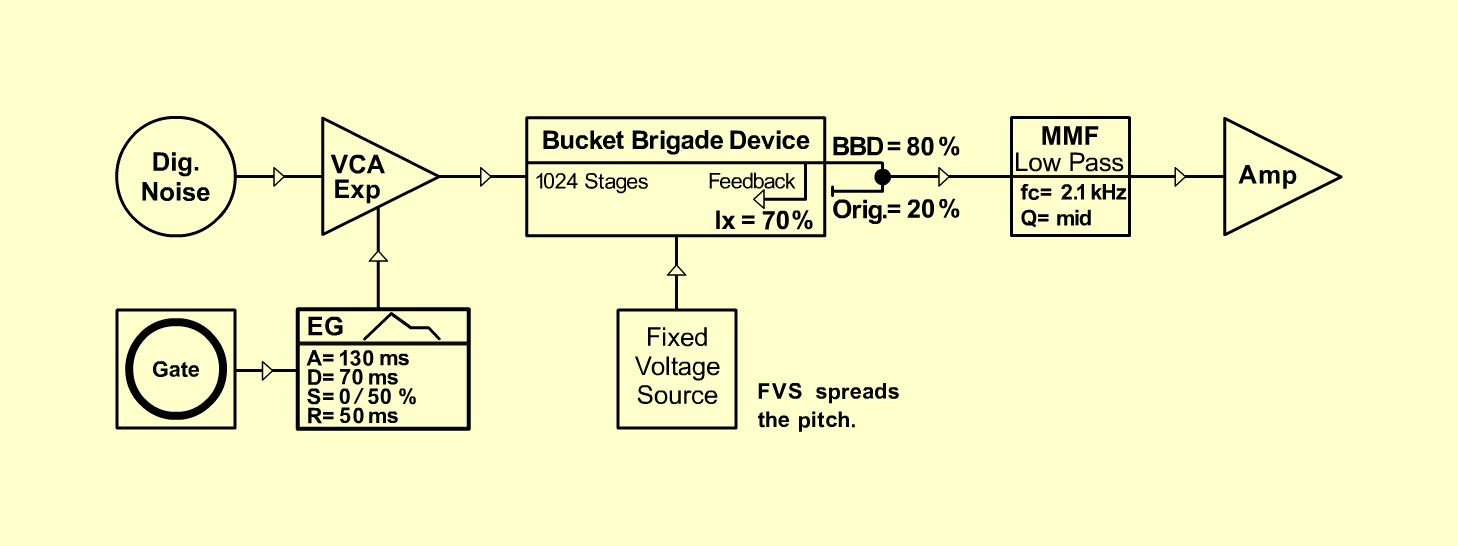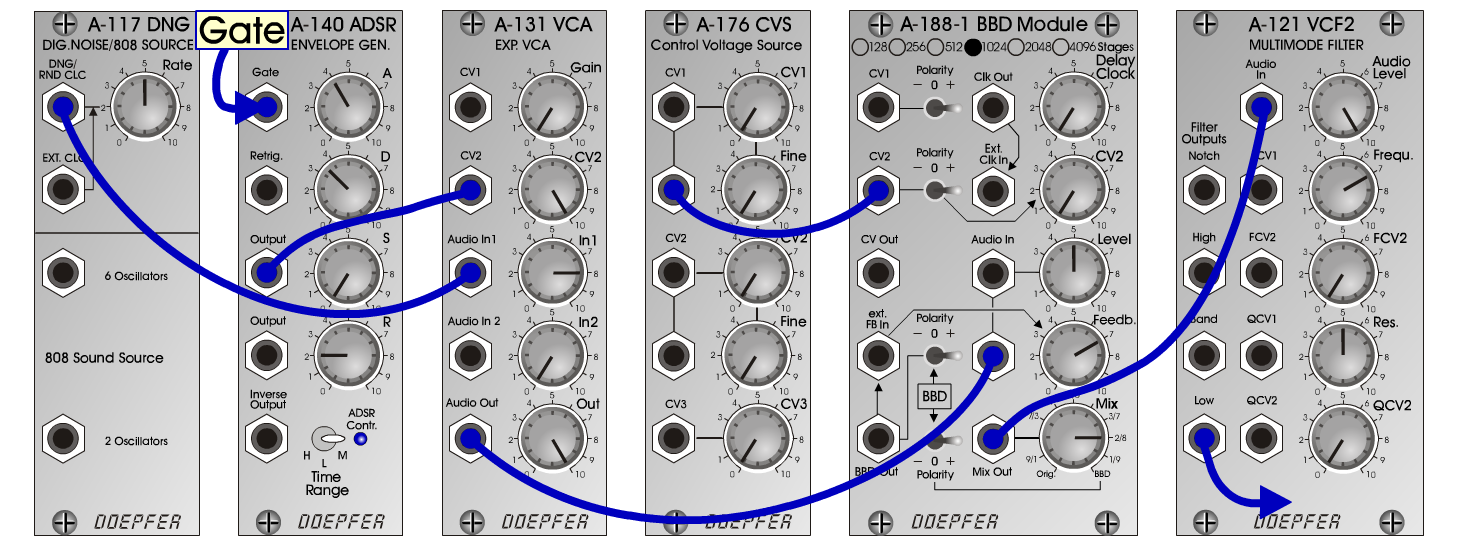Oberon-BottleInstrumentsD o e p f e r A - 100 Connections: Settings: A-117 (DNG) <=> A-131 (Audio In) A-131 (Audio Out) <=> A-188-1 (Audio In) Gate <=> A-140 (Gate) A-140 (Output) <=> A-131 (CV2) A-176 CVS (CV 1) <=> A-188-1 (CV2) A-188-1 (Mix Out) <=> A-121 (Audio In) A-121 (Low) <=> Amp A-117 (Rate = 5) A-188-1 (Level = 5, Feedb. = 7, Mix = 2/8, Polarity = +) A-121 (Audio Level = 10, Freq = 7, Res = 5) A-140 (A =4 , D = 3.5, S = 0, R = 2, Range = M) A-131 (Gain = 0, CV2 = 10, Audio In 1 = 8, Audio Out = 10) Spread the pitch:  A-188-1 (Delay/Clock) and  A-188-1 (CV 2) BottleL: A-140 (S = 5) Josef MuellerSound samples Oberon-Bottle-Piece (Theme: Bach - BWV 1080) Oberon-Bottle short (130 Hz) Oberon-Bottle short (261 Hz) Oberon-Bottle long (130 Hz) Oberon-Bottle long (261 Hz)